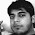### Minimum Point in a Rotated Sorted Array

Source: Asked for a data scientist position at a technology startup in financial services sector in London

Problem:

What is the optimal algorithm to find the minimum element given a rotated sorted array of integers?
A rotated sorted array of integers is the output array of a rotation operation performed on a sorted array.
Eg: 3 5 6 1 2

Update (24 June 2014):
Solution:
Posted by Gaurav Bijay Kumar (CSE IITB 2006, Goldman Sachs Quant, Morgan Stanley Quant, Chicago Booth MBA, Credit Suisse IB, Goldman Sachs Strat), Sandeep, Khalil Sawant, Himanshu, ciddhi and gaurushh.

1.O(lg n)

FindMin( A )

If (n==1)
return A(0);
else
{
L = A[ 0 ... n/2-1 ];
R = A[ n/2 ... n-1 ];

if( L(0) <= L(n/2-1) and R(n/2) >= R(n-1) )
return FindMin( R );
else
return FindMin( L );
}

1.Yo. Awesome. Thanks

2.It will not work for duplicate entries.
Example
11101111111

3.Its not working for
5,6,7,8,9,10,1,2,3

2.If the array is sorted in increasing order then the minimum element is always the element next to the maximum (unless rotation is from starting element in which case max is last and min is first). Upon any other single rotation, the array is divided to two continuous and non decreasing parts such that all elements of the second part (i.e. with indices greater than first) are less than first lets call them A and B respectively. Now implement a traversal like binary search wherein we try to find the min element. Its the first element in B. At any position i, if F(i)>F(0) then its in A else in B. Using this idea, we discard portions of the array. We also check if F(i)>F(i-1). Once this is violated, we stop. The complexity is, as can be trivially seen, logarithmic in number of elements.

1.3.If the array was sorted
arr[start] <= arr[middle] <= arr[end]

Since the array is rotated, once of the above two conditions is violated

For the half that the condition is violated, repeat the iteration on that half of array

Recurse

1.4.This can be done in O(log(n)) using a binary search for the first element x that's smaller than it's previous element

1.Your solution needs to be elaborated. Thanks

5.take --> low -- mid -- high
if a[low]> a[mid] then
high=mid and find new mid
if a[low] < a[mid]
log = mid and find new mid

this is the basic algo, some special cases need to be added.

1.6.int minPoint(int a[],int n)
{
int mid=n/2;
if(n==1)
return 0;
if(a[mid-1]>a[mid])
return mid;
if(a[mid]>a[mid+1])
return mid+1;
if(aa[n-1])
return mid+minPoint(a+mid+1,n-mid-1);
else
return minPoint(a,mid);
}

1.Other than a few mistakes in the code, in principal the solution looks correct. Thanks

7.It can be done using binary search algorithm in O(logn).
Split at mid point and check the first and last element of both the partitions. We go further in the one which has A[first element]>A[last element] (The order is opposite as should be as per the sorting).

1.Lets say if it is in increasing order, then the algo is:
You compare a[n/2-1] and a. If a[n/2-1] is bigger, then you compare a with middle element of the partial array (a[n/2-1] to a[end]), else
you compare with the middle element of the partial array (a to a[n/2-1]).
You continue like this until you have left with no space to move right or left. You will ultimately fall on the smallest.
In case of decreasing order, just switch the direction of movement.
Although this needs additional information of increasing or decreasing to make movements.

2.Thanks ciddhi and gaurushh.